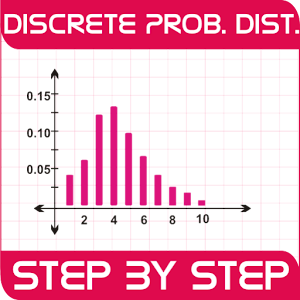# Discrete Probability Dist. (P) - Lumos Educational App Store1
Price -NA
\$A

#### DESCRIPTION:

*** Discrete Probability Distribution (Pro) *** Discrete Probability Distribution App is a unique and interactive statistical tool to learn theory and concepts of Discrete Probability Distributions. Probability distribution assigns a probability to the event that a random variable,survey falls within specified range of continuous value. It is developed and designed by qualified Statistics Expert. It is designed in such a way that user can learn theory step by step, and use the theoretical concepts to solve numerical problems using calculation and step by step dynamic reporting. U

#### OVERVIEW:

Discrete Probability Dist. (P) is a free educational mobile app By NovelAnswer.It helps students in grades 8 practice the following standards .

This page not only allows students and teachers download Discrete Probability Dist. (P) but also find engaging Sample Questions, Videos, Pins, Worksheets, Books related to the following topics.

8